Python数据分析：基于Pandas数据清洗2021-04-06 17:00:261523浏览 · 0收藏 · 0评论

1.导入方法read_excel

```# 导入数据
import pandas as pda
import matplotlib.pylab as pyl

a = pda.read_excel("D:\\迅雷下载\\工具\\表格\\练习.xls")	# 路径使用双反斜杠，否则会报错
print(len(a))   # 数据框的长度，是按行统计的
123456```

2.发现缺失值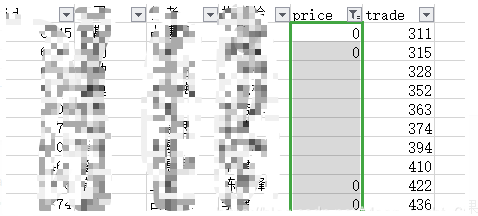```b = ["price", "trade"]
for i in b:
a[i][(a[i] == 0)] = None
# a["price"] == 0  判断语句，返回True或False  ,对列表的每一个值进行判断，如果有0，该处值置为none，然后进行判断直至完成
1234```

3.缺失值处理

```x = 0
for j in b:
for k in range(len(a)):
if (a[j].isnull())[k]:
a[j][k] = 36
x += 1
print(x)```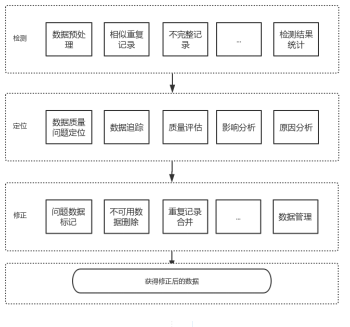（推荐操作系统：windows7系统、Python 3.9.1，DELL G3电脑。）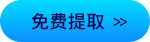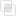Python数据分析 | pandas汇总和计算描述统计python数据分析的一般步骤是什么python数据分析学什么书python数据分析能做什么python3中json编码是什么？怎么用？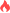1209